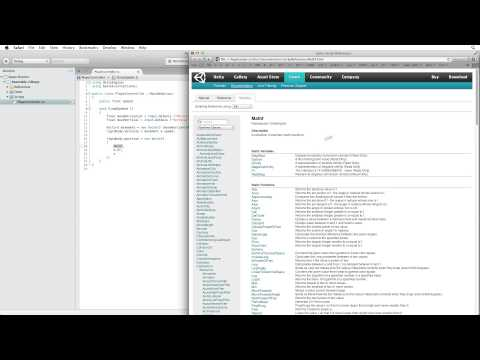Moving the player

難易度: 初級

Writing simple C# code to capture player input and move the player ship.Moving the player

トランスクリプト

Please note that this project was developed for Unity version 4. It currently works, and has been checked, with Unity version 5.1. Please turn annotations on while watching the video, as we can add annotations to the videos when there are discrepancies between the recorded content and the current version of Unity.

Get the Upgrade Guide for Unity 5 here.

Please refer to the Official Q&A Page on the Forums for an Upgrade FAQ and to ask any questions.

Code snippet

``````using UnityEngine;
using System.Collections;

[System.Serializable]
public class Boundary
{
public float xMin, xMax, zMin, zMax;
}

public class PlayerController : MonoBehaviour
{
public float speed;
public float tilt;
public Boundary boundary;

void FixedUpdate ()
{
float moveHorizontal = Input.GetAxis ("Horizontal");
float moveVertical = Input.GetAxis ("Vertical");

Vector3 movement = new Vector3 (moveHorizontal, 0.0f, moveVertical);
rigidbody.velocity = movement * speed;

rigidbody.position = new Vector3
(
Mathf.Clamp (rigidbody.position.x, boundary.xMin, boundary.xMax),
0.0f,
Mathf.Clamp (rigidbody.position.z, boundary.zMin, boundary.zMax)
);

rigidbody.rotation = Quaternion.Euler (0.0f, 0.0f, rigidbody.velocity.x * -tilt);
}
}``````
``````class Boundary
{
var xMin : float;
var xMax : float;
var zMin : float;
var zMax : float;
}

var speed : float;
var tilt : float;
var boundary : Boundary;

function FixedUpdate () {
var moveHorizontal : float= Input.GetAxis ("Horizontal");
var moveVertical : float= Input.GetAxis ("Vertical");

var movement : Vector3= new Vector3 (moveHorizontal, 0.0f, moveVertical);
rigidbody.velocity = movement * speed;

rigidbody.position = new Vector3
(
Mathf.Clamp (rigidbody.position.x, boundary.xMin, boundary.xMax),
0.0f,
Mathf.Clamp (rigidbody.position.z, boundary.zMin, boundary.zMax)
);

rigidbody.rotation = Quaternion.Euler (0.0f, 0.0f, rigidbody.velocity.x * -tilt);
}``````
``````import UnityEngine
import System.Collections

[System.Serializable]
public class Boundary:
public xMin as single
public xMax as single
public zMin as single
public zMax as single

public class PlayerController(MonoBehaviour):
public speed as single
public tilt as single
public boundary as Boundary

private def FixedUpdate():
moveHorizontal as single = Input.GetAxis('Horizontal')
moveVertical as single = Input.GetAxis('Vertical')

movement = Vector3(moveHorizontal, 0.0F, moveVertical)
rigidbody.velocity = (movement * speed)
rigidbody.position = Vector3(Mathf.Clamp(rigidbody.position.x, boundary.xMin, boundary.xMax), 0.0F, Mathf.Clamp(rigidbody.position.z, boundary.zMin, boundary.zMax))
rigidbody.rotation = Quaternion.Euler(0.0F, 0.0F, (rigidbody.velocity.x * (-tilt)))``````

関連ドキュメント